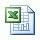# Maximum surface pressure from kick tolerance factor

You have learned about the kick tolerance factor from the previous blog posting and this article will demonstrate you how to use the kick tolerance factor to determine the maximum surface pressure from it.

The formula is listed below;

Maximum surface pressure = kick tolerance factor x 0.052 x TVD of well

Where;

Maximum surface pressure in psi

kick tolerance factor in ppg

TVD of well in ft

Determine the maximum surface pressure with this following data:

Kick tolerance factor = 1.8 ppg

Hole depth = 10,000’MD/9500’TVD

Based on these data, you can calculate the maximum surface pressure like this;

Maximum surface pressure = 1.8 x 0.052 x 9500 = 889 psi

Please find the Excel sheet for this calculationMaximum surface pressure from kick tolerance factor

Share the joy1.George B. Heckler says: